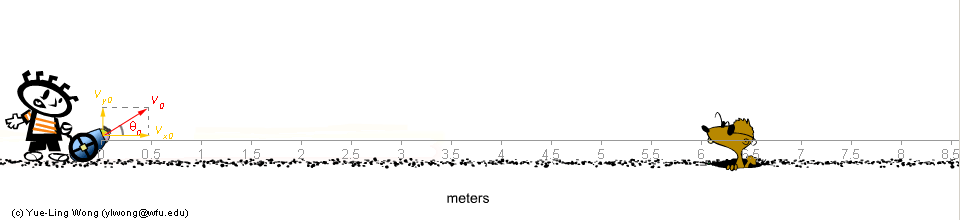Science Education Tutorials and Practice Exercises

 1H NMR 13C NMR Chemical Tests Functional Group Identification

General Physics :: Projectile Motion :: How to Calculate #1Problem #1: To find out where the cannon ball will land, given the initial velocity ( v0) and the firing angle (θ0)
(The parameters whose values are known are highlight in red. The main direction of solving a problem: try to derive equations that use these parameters with known values.)

Solution Step-by-step:

1. Resolve the initial velocity vector into x and y components.
Initial velocity:
 x-component: vx0 = v0 × cos θ0 y-component: vy0 = v0 × sin θ0

 Step 1 Calculate: vx0 and vy0 From: v0 and θ0 Try it: Change the values for v0 and θ0 in the equations below, and then click the Calcuate button to calcuate the vx0 and vy0: vx0 = v0 × cos θ0 vx0 = m/sec. × cos (degree) = 6.928 m/sec. vy0 = v0 × sin θ0 vy0 = m/sec. × sin (degree) = 4 m/sec.

2. Analyze the horizontal and vertical motion independently:
• Vertical motion: make use of the constant acceleration (g)
vy = vy0 - g × t

where g is gravity (9.8 m/sec.) or free-fall acceleration, and t is the time elapsed.

 When the object reaches the top of its parabola motion path, vy = 0. i.e.      vy0 - g × tmax = 0 Þ      tmax = vy0 / g where tmax is the time it takes for the object to reach the maximum height.

 Step 2 Calculate: tmax From: vy0 Try it: Use the values of vy0 obtained above to calculate the time (tmax) when the object reaches its maximum height: tmax = vy0 / g tmax = m/sec. / 9.8m/sec. = 0.408 sec.

So, now you know how long it takes for the object to reach the top of its parabola motion path. It will start falling down after this moment.

What is finding out this timing to do with finding out where the cannon ball will land and the horizontal location when it reaches the highest point?

Because of the symmetry of the vertical motion, it takes the same amount of time for the cannon ball to return to the ground (assume the cannon ball was fired at the ground level). Thus, the whole journey of the cannon ball, from firing to returning to the ground, takes:

 ttotal = 2 × tmax

 Step 3 Calculate: ttotal From: tmax Try it: Use the tmax obtained above to calculate the total time (ttotal) for the cannon ball to return to the ground: ttotal = 2 × tmax ttotal = 2 × sec. = 0.816 sec.

For example, if it takes 3 seconds for the cannon ball to reach its highest point in its parabola motion path, then it takes another 3 seconds for it to come back from the highest point. The total time for the cannon ball to return to the ground after firing is 6 seconds.

This timing information can be used to calculate where the cannon ball will land. (See the horizontal motion analysis below)

• Horizontal motion: make use of the constant velocity (vx)
vx = vx0

the horizontal distance (d x) the cannon ball has traveled when it lands on the ground:

 d x = vx0 × ttotal

 Step 4 Calculate: dx From: vx0 and ttotal Try it: Use the ttotal obtained above to calculate the dx: dx = vx0 × ttotal dx = m/sec. × sec. = 5.653 m

[an error occurred while processing this directive]